Courses

# Ampere's Theorem - Magnetism, Electromagnetic Theory, CSIR-NET Physical Sciences Physics Notes | EduRev

## Physics for IIT JAM, UGC - NET, CSIR NET

Created by: Akhilesh Thakur

## Physics : Ampere's Theorem - Magnetism, Electromagnetic Theory, CSIR-NET Physical Sciences Physics Notes | EduRev

The document Ampere's Theorem - Magnetism, Electromagnetic Theory, CSIR-NET Physical Sciences Physics Notes | EduRev is a part of the Physics Course Physics for IIT JAM, UGC - NET, CSIR NET.
All you need of Physics at this link: Physics

Ampere's Law

The magnetic field in space around an electric current is proportional to the electric current which serves as its source, just as the electric field in space is proportional to the chargewhich serves as its source. Ampere's Law states that for any closed loop path, the sum of the length elements times the magnetic field in the direction of the length element is equal to the permeability times the electric current enclosed in the loop.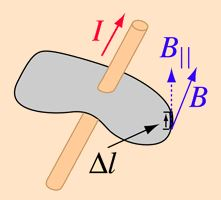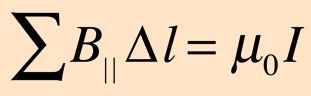In the electric case, the relation of field to source is quantified in Gauss's Law which is a very powerful tool for calculating electric fields.

Ampere's Law Applications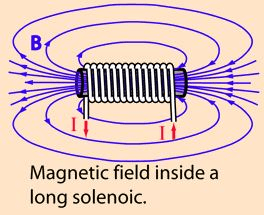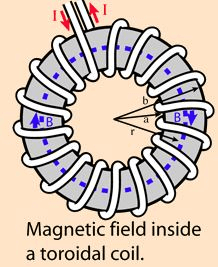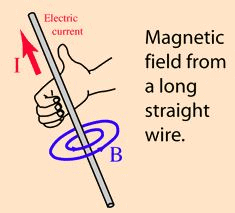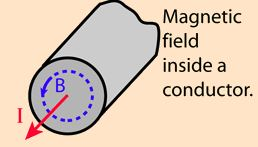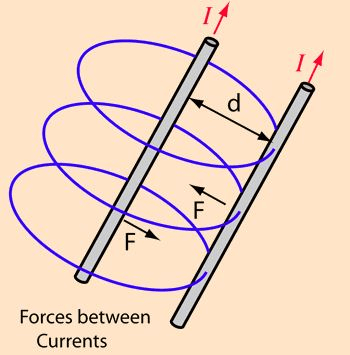Solenoid Field from Ampere's Law

Taking a rectangular path about which to evaluate Ampere's Law such that the length of the side parallel to the solenoid field is gives a contribution BL inside the coil. The field is essentially perpendicular to the sides of the path, giving negligible contribution. If the end is taken so far from the coil that the field is negligible, then the length inside the coil is the dominant contribution.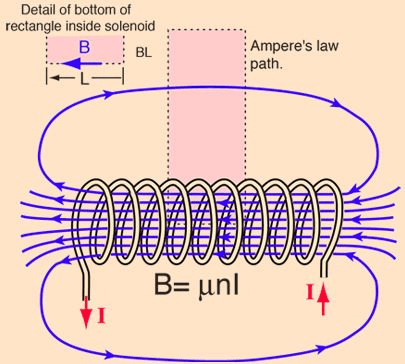This admittedly idealized case for Ampere's Law gives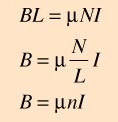This turns out to be a good approximation for the solenoid field, particularly in the case of an iron core solenoid

Magnetic Field of Toroid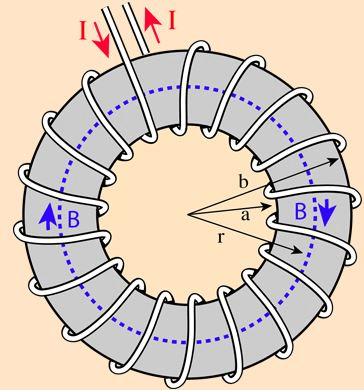Finding the magnetic field inside a toroid is a good example of the power of Ampere's law. The current enclosed by the dashed line is just the number of loops times the current in each loop. Amperes law then gives the magnetic field by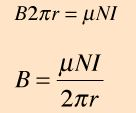The toroid is a useful device used in everything from tape heads to tokamaks

Magnetic Field of Current

The magnetic field lines around a long wire which carries an electric current form concentric circles around the wire. The direction of the magnetic field is perpendicular to the wire and is in the direction the fingers of your right hand would curl if you wrapped them around the wire with your thumb in the direction of the current.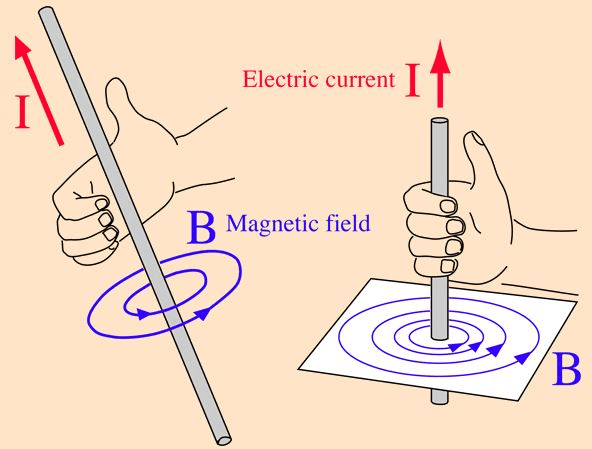Magnetic Field Inside a Conductor

The magnetic field inside a conductor with uniform current density J = I/πR2 can be found with Ampere's Law.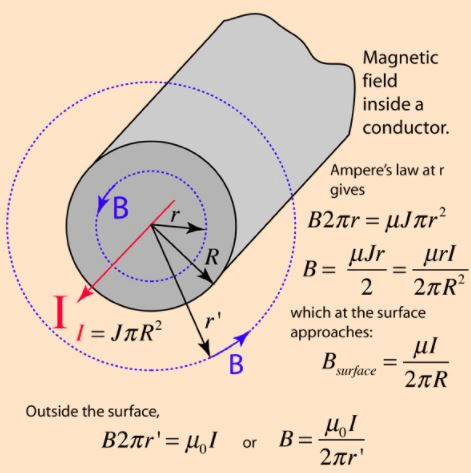Inside the conductor the magnetic field B increases linearly with r. Outside the conductor the magnetic field becomes that of a straight conductor and decreases with radius. Note that the expressions for inside and outside would approach the same value at the surface if the magnetic permeability were the same.

Magnetic Force Between Wires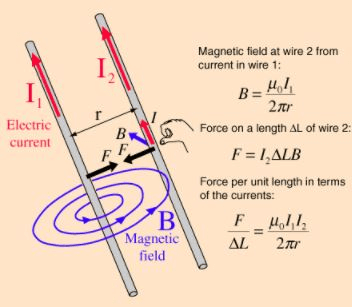The magnetic field of an infinitely long straight wire can be obtained by applying Ampere's law. The expression for the magnetic field is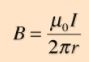Once the magnetic field has been calculated, the magnetic force expressioncan be used to calculate the force. The direction is obtained from the right hand rule. Note that two wires carrying current in the same direction attract each other, and they repel if the currents are opposite in direction. The calculation below applies only to long straight wires, but is at least useful for estimating forces in the ordinary circumstances of short wires. Once you have calculated the force on wire 2, of course the force on wire 1 must be exactly the same magnitude and in the opposite direction according to Newton's third law.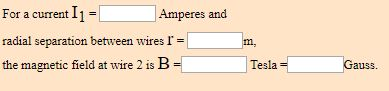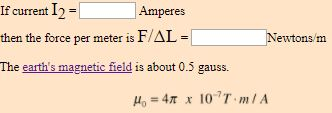Offer running on EduRev: Apply code STAYHOME200 to get INR 200 off on our premium plan EduRev Infinity!

159 docs

,

,

,

,

,

,

,

,

,

,

,

,

,

,

,

,

,

,

,

,

,

,

,

,

,

,

,

;